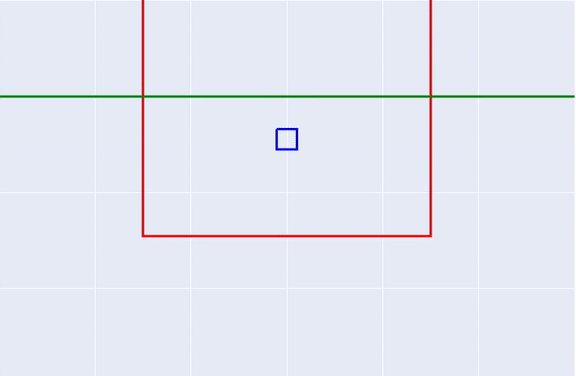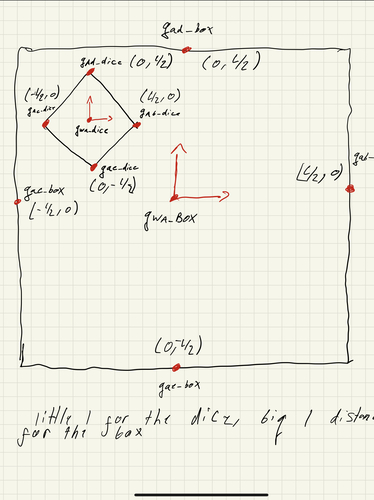## Physics Simulation### Project information

The goal of this project was to demonstrate modeling a dynamic simulation using Lagrangian dynamics. This project is meant to simulate the physics of 2 cups, one inside of another, when a force is exerted. The main outcome of the project was my ability to understand how these simulations work, which will allow me in the future to better judge the accuracy of a simulation.

The system has 6 degrees of freedom. This means there are 6 configuration variables, 3 for each box. Each object is fully defined with an x and y coordinate and an angle (θ).

There are 16 constraints because each side of the jack can hit any of the four walls of the box. I formed the constraint equations by calculating the position of tips of the jack in the frames of the box walls. Then, I set the x or y coordinate of the transformation matrix equal to 0 representing collision between the jack and the box. For the transformations between the jack and the left and right walls of the box, I set the x coordinate equal to zero. For the transformations between the jack and the top and bottom walls of the box, I set the y coordinate equal to zero.